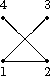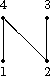Recursion and orderly generation

## Recursion and orderly generation

Besides this direct method of evaluating a transversal of the symmetry classes with prescribed content, there exists also a recursive method, where the recursion is on the cardinality of the range. We are going to describe this next and then we shall combine it with the so-called orderly generation. It is based on the following observation:

Lemma: Assume an action GX, and that BÍX is a block or, as it is sometimes called a system of imprimitivity (which means that B is mapped under gÎG either onto B or onto a subset gB which is disjoint with B). Then the following is true:
• For each orbit wÎG\\X we have
wÇB not =Æ iff wÇgB not =Æ,
which means that B and gB intersect with the same orbits.
• For each bÎB, gÎG, gbÎB is true if and only if
gÎNG(B):={gÎG | gB=B}.
Therefore, if
X=ÈiÎIBi,
a disjoint union of blocks, and JÍI such that
T(G\\{Bi | iÎI})={Bj | jÎJ}
is a transversal of G on the set of these blocks, then a transversal T(G\\X) is the following union of transversals:
T(G\\X)=ÈjÎJT(NG(Bi)\\Bi),
where T(NG(Bi)\\Bi) is an arbitrary transversal of NG(Bi)\\Bi.

The checks are easy and left as an exercise. This result shows that the direct evaluation of a transversal can be replaced by successive evaluations of blocks, their normalizers and transversals of the orbits of the normalizers on the blocks. An interesting method systematically to obtain blocks can be derived from

The Homomorphism Principle Assume that G acts on the sets Xi, i=1,2, and that j:X2 -> X1 commutes with the action:
j(gx2)=gj(x2), for each gÎG,x2ÎX2,
then each inverse image j-1(x1),x1ÎX1, is a block. Moreover, the normalizer of this block is the stabilizer of x1:
NG(j-1(x1))=Gx1.

This is easy to check but very important to remark since it is essential for

Surjective Resolution Assume that G acts on the sets Xi,iÎm, and that we are given surjective mappings
ji+1:Xi+1 -> Xi, for each iÎm-1,
that commute with the action:
ji+1(gxi+1)=gji+1(xi+1), for each iÎm-1, gÎG,xi+1ÎXi+1.
Then we can obtain a transversal T of G\\Xm by successively working backwards, starting by evaluating a transversal of G\\X1 and the stabilizers of its elements, and then evaluating the inverse images j2(x) of the elements x in this transversal, as well as the transversals Gx\\j2-1(x), and so on, in accordance with the lemma.

We would like now to apply this to symmetry classes in order to obtain a recursive evaluation of a transversal, using recursion on | Y | .

Example: For sake of simplicity we assume that
YX=mn,
and that G acts on n and so on YX=mn in the canonic way. The Surjective Resolution method can be applied since the following mapping commutes with the action of G on the G-sets kn:
jk:kn -> (k-1)n:f -> f',
where f' is defined by
f'(i):=f(i) if f(i)Îk-1
f'(i):=k-1 otherwise.
We can therefore start from X1:=1n, which consists of the single and constant mapping i -> 1, iÎn. The stabilizer is G, and the inverse image is the whole set X2:=2n. We therefore have to evaluate in this second step a transversal T2 of G\\2n. Assume that this is done, and let f2 denote an element of T2, Gf2 its stabilizer. It remains to evaluate in the third step, for each such f2 and its stabilizer a transversal T3 of
Gf2\\j3-1(f2).
This inductive step can be carried out as follows. Two elements f3 and f3' of j3-1(f2) can differ only on the inverse image f2-1(2) of the point 2, and the values there can only be 2 or 3. We therefore need only to evaluate a transversal T of
Gf2\\{2,3}f2-1(2).
The desired transversal T3 then consists of the mappings f3"Î3n such that
f3" | f2-1(2)ÎT, and f3" | n\f2-1(2)=f2 | n\f2-1(2).

The method of Surjective Resolution can be combined with the method of orderly generation as described by R. C. Read. This way of generation is based on the fact that total orders on X and Y induce a canonic total order on YX, so that a canonic transversal

T>(G\\YX),

consisting of the biggest elements of the orbits does exist. Moreover, we can break this problem into pieces, if weights are at hand.

Example: Recall that the graphs on v vertices correspond to the set of orbits
Sv\\2v choose 2.
As v is totally ordered, so is the set of pairs v choose 2, and hence also the set of labelled graphs. This is in fact the lexicographic order £ on the set of 0-1-sequences obtained from the adjacency matrices by reading the upper triangular part row by row from left to right and from top to bottom. For exampleis mapped onto the sequence (1,1,0,0,1,0), and hence this labelled graph is a member of the canonic transversal, but (0,0,1,1,1,0), which corresponds to the isomorphic labelled graphis not a canonic representative. The problem of orderly generation now means the following. We would like to find an economic way of generating 0-1-sequences of length 6 in such a way that not too many sequences are generated, which do not correspond to the desired canonic representatives. Moreover, we should like to do this by increasing the number of ones successively by 1, which means by successively increasing the number of edges. One way of doing this is to start off from the sequence (0,0,0,0,0,0), which forms the canonic transversal of the labelled graphs of total weight 0:
T>(0)={(0,0,0,0,0,0)}.
Now we will evaluate T>(1), then T>(2), and so on, in the following way. Assume that T>(i) is at hand. Then, in order to obtain T>(i+1), we shall apply to each member of T>(i) the following augmentation. We change in each element of T>(i) in all the possible ways just one entry zero that is to the right of the rightmost entry 1. The resulting set of labelled graphs is either empty, if no such entry exists, or it consists of labelled graphs with one more edge. These sets have to be checked carefully, the canonic sequences have to be kept, the others have be thrown away before going to the next step. The remaining set of labelled graphs is in fact the desired transversal T>(i+1). In our example this process runs as follows:
• [1.] In the first step we get the six sequences containing exactly one entry 1. The canonic respresentative is, of course, (1,0,0,0,0,0), and so, clearly
T>(1)={(1,0,0,0,0,0)}.
• [2.] In the second step we start with (1,0,0,0,0,0) and obtain, after canceling the sequences which are not canonic, that
T>(2)={ (1,1,0,0,0,0),(1,0,0,0,0,1)}.
• [3.] The third step gives, from the first canonic representative of the second step, the sequences (1,1,1,0,0,0), (1,1,0,1,0,0) and (1,1,0,0,0,1), while the other representative of T>(2) needs not to be considered, as there is no zero entry to the right of the rightmost entry 1. Thus
T>(3)={(1,1,1,0,0,0),(1,1,0,1,0,0),(1,1,0,0,0,1)}.
• [4.] In the fourth step we get (1,1,1,1,0,0), from the first element of T>(3), nothing new from the second one, but (1,1,0,0,1,1) from the third element, and hence
T>(4)={(1,1,1,1,0,0),(1,1,0,0,1,1)}.
• [5.] The next step gives
T>(5)={(1,1,1,1,1,0)},
from which we obtain in
• [6.] the final step that
T>(6)={(1,1,1,1,1,1)}.
And so, summing up, we have obtained the desired canonic transversal of the isomorphism classes of labelled graphs on 4 vertices:
T>={(0,0,0,0,0,0),(1,0,0,0,0,0),(1,1,0,0,0,0),
(1,0,0,0,0,1), (1,1,1,0,0,0),(1,1,0,1,0,0),(1,1,0,0,0,1),
(1,1,1,1,0,0),(1,1,0,0,1,1),(1,1,1,1,1,0), (1,1,1,1,1,1)}.

It is important to note that we had to check 24 sequences for canonicity, out of 26=64 labelled graphs on 4 vertices, which is not bad. Moreover, we note that each of the canonic representatives arises just once from an element of smaller total weight. The corresponding generalization reads as follows:

Theorem: (Read) Assume a finite action GX where X is totally ordered by £ and a disjoint union
X=Èni=1Xi,
of invariant and nonempty subsets Xi. Let A denote an algorithm that produces for each xÎX either the empty set or a set A(x)ÍX in descending order, such that the following conditions hold for the canonic transversals T>(i) of the orbit sets G\\Xi, for each iÎn-1:
• T>(i+1)ÍÈA(x), union over the xÎT>(i)},
• for each xÎT>(i+1) there exists a unique element x'ÎT>(i) such that xÎA(x'), and finally,
• for any x1,x2ÎT>(i+1), x1<x2, we have the implication
x1ÎA(x'1),x2ÎA(x'2) imply x'1<x'2.
Then, computing T>(1) and recursively running through T>(i) with x, producing the augmentation A(x), and eliminating representatives which are not canonic from A(x), for iÎn-1, gives the desired canonic transversal T> of G\\X as the following union:
T>=Èi=1nT>(i).

This orderly generation obviously will work as soon as we have found an augmentation procedure A that has the properties listed in the items of the above theorem of Read. We already saw such an augmentation that can be used for the graphs and which amounts to adding an edge in a certain set of places. The necessary canonicity test can be done quite efficiently using any suitable invariant that allows to put the canonic representatives in what is called an AVL-tree in computer science. For example, in the molecular graphs case, we can use a so-called Morgan table, which is a numbering well known to chemists, and used in the coding procedure for chemical substances in the Chemical Abstracts. It reduces the set of checks to a reasonable small number.

Summing up we should like to mention that an efficient way of evaluating a transversal of the symmetry of G on YX can be implemented by using the following methods:

• A recursion procedure, using recursion on | Y | .
• Read's orderly generation, by applying lexicographic or any other ordering of YX.
• A canonic form of the elements fÎYX which allows to put the canonic representatives (canonic with respect to the ordering mentioned in the second item) together into an AVL-tree and which reduces the necessary number of checks to a reasonably small one.
Exercises
E: Check the lemma.

 harald.fripertinger "at" uni-graz.at http://www-ang.kfunigraz.ac.at/~fripert/ UNI-Graz Institut für Mathematik UNI-Bayreuth Lehrstuhl II für Mathematik
last changed: January 19, 2005Recursion and orderly generation